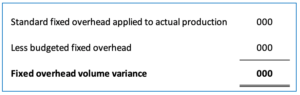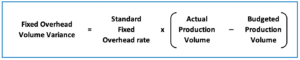## Overview

Fixed overhead volume variance is the difference between the budgeted fixed overhead cost and the fixed overhead costs that are applied to the actual production volume using the standard fixed overhead rate. Likewise, the company can calculate the fixed overhead volume variance with the formula of comparing fixed overhead costs that are applied to the actual production to the budgeted fixed overhead after determining the standard fixed overhead rate.

If the fixed overhead cost applied to the actual production using the standard fixed overhead rate is bigger than the budgeted fixed overhead cost, the fixed overhead volume variance is the favorable one. This means that the company’s actual production volume measured in units or hours during the period is more than the budgeted production volume that the company has previously planned.

On the other hand, if the budgeted fixed overhead cost is bigger instead, the result will be unfavorable fixed overhead volume variance. This means that the actual production volume is lower than the planned or scheduled production.

It is a bit confusing if we only look at the dollar amount. However, as the name suggested, it is the fixed overhead volume variance that is more about the production volume. Likewise, we can also determine whether the fixed overhead volume variance is favorable or unfavorable by simply comparing the actual production volume to the budgeted production volume.

If the actual production volume is higher than the budgeted production, the fixed overhead volume variance is favorable. On the other hand, if the actual production volume is lower than the budgeted one, the variance is unfavorable.

Actual production volume > Budgeted production volume => Favorable variance
Actual production volume < Budgeted production volume => Unfavorable variance

The budgeted production volume here is also referred to as the normal capacity of the company or the existing facility in the production. Likewise, if the actual production exceeds the normal capacity, the result is favorable fixed overhead volume variance and vice versa.

## Fixed overhead volume variance formula

The company can calculate fixed overhead volume variance with the formula of standard fixed overhead applied to actual production deducting the budgeted fixed overhead.Standard fixed overhead applied to actual production is the fixed overhead cost that is applied to the actual production volume using the standard fixed overhead rate.

Standard fixed overhead applied to actual production = standard fixed overhead rate x actual production volume

Standard fixed overhead rate can be calculated with the formula of budgeted fixed overhead cost dividing by the budgeted production volume.

Standard fixed overhead rate = Budgeted fixed overhead cost / Budgeted production volume

Alternatively, the fixed overhead volume variance formula can be broken down to the standard fixed overhead rate multiplying with the difference between the actual and budgeted production volume as below:

Fixed overhead volume variance formula (2):Actual production volume is the production that the company actually achieves (in hours) or produces (in units) during the period. The figure in hours here can either be labor hours or machine hours depending on which one is more suitable for the measurement in the production.

On the other hand, the budgeted production volume is the production volume that the company estimates to produce or achieve during the period. It is the normal capacity that the company or the existing facility can achieve for the period. This figure is usually included in the budget of production that is planned or scheduled before the production starts.

## Fixed overhead volume variance example

For example, the company ABC has the following budgeted fixed overhead costs for the month of August as extracted from the budget plan for production:

Salaries of manager and supervisors \$8,000
Depreciation \$6,000
Other costs \$5,000

In August, the company ABC which is a manufacturing company has produced 950 units of goods in the production. However, the company ABC has the normal capacity of 1,000 units of production for August as they are scheduled to produce in the budget plan.

What is fixed overhead volume variance in August? Is it favorable or unfavorable?

Solution:

With the information in the example, the company ABC can calculate the fixed overhead volume variance in August with the formula below:

Fixed overhead volume variance = Standard fixed overhead rate x (Actual production volume – Budgeted production volume)

Standard fixed overhead rate = \$19,000 / 1,000 units = \$19 per unit

Fixed overhead volume variance = \$19 x (950 units – 1,000 units)

Fixed overhead volume variance = \$18,050 – \$19,000 = \$950 (U)

As a result, the company has an unfavorable fixed overhead variance of \$950 in August. This is due to the actual production volume that it has produced in August is 50 units lower than the budgeted one.

This result of \$950 of unfavorable fixed overhead volume variance can be used together with the fixed overhead budget variance to determine the total fixed overhead variance.

Alternatively, we can use the first formula of fixed overhead volume variance which would yield the same result as below:

 Standard fixed overhead applied to actual production (\$19 x 950 units) \$18,050 Less budgeted fixed overhead (\$19,000) Fixed overhead volume variance \$950 (U)